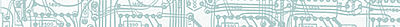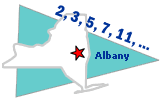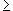# Negation of a StatementExample 1:  Examine the sentences below.1 Every triangle has three sides. 2 Albany is the capital of New York State. 3 No prime number is even.Each of these sentences is a closed sentence.

Definition: A closed sentence is an objective statement which is either true or false.

Thus, each closed sentence in Example 1 has a truth value of either true or false as shown below.

 1 Every triangle has three sides. true 2 Albany is the capital of New York State. true 3 No prime number is even. false

Note that the third sentence is false since 2 is a prime number. It is possible that a closed sentence will have different truth values at different times. This is demonstrated in Example 2 below.

Example 2:

 1 Today is Tuesday. 2 Bill Clinton was the 42nd President of the United States.Example 3: Examine the sentences below.

 1 x + 3 = 7 2 She passed math. 3 y - 4 = 11 4 He is my brother.

The sentences in Example 3 are open sentences.

Definition: An open sentence is a statement which contains a variable and becomes either true or false depending on the value that replaces the variable.

Let's take another look at Example 3.  This time we will Identify the variable for each open sentence.

 1 x + 3 = 7 The variable is x. 2 She passed math. The variable is she. 3 y - 4 = 11 The variable is y. 4 He is my brother. The variable is he.

Now that we have identified the variables, we can analyze the meaning of these open sentences. Sentence 1 is true if x is replaced by 4, but false if x is replaced by a number other than 4. Sentence 3 is true if y is replaced by 15, but false otherwise. Sentence 2 is either true or false depending on the value of the variable "she." Similarly, sentence 4 is either true or false depending on the value of the variable "he." In summary,  the truth value of each open sentence depends on what value is used to replace the variable in that sentence.

Example 4:

 Given: Let p represent, "Baseball is a sport." Let q represent, "There are 100 cents in a dollar." Let r represent, "She does her homework." Let s represent, "A dime is not a coin." Problem: Write each sentence below using symbols and indicate if it is true, false or open.
 1 A dime is a coin. ~s true 2 Baseball is not a sport. ~p false 3 She does her homework. r open 4 There are not 100 cents in a dollar. ~q false 5 She does not do her homework. ~r open 6 Baseball is a sport. p true

Example 5:

 Given: Let p represent the closed sentence "The number 9 is odd." Problem: What does ~p mean?

In Example 5 we are asked to find the negation of p.

Definition: The negation of statement p is "not p."  The negation of p is symbolized by "~p."  The truth value of ~p is the opposite of the truth value of p.

Solution: Since p is true, ~p must be false.

 p: The number 9 is odd. true ~p: The number 9 is not odd. false

Let's look at some more examples of negation.

Example 6:

 r: 7 < 5 false ~r: 75 true

Example 7:

 a: The product of two negative numbers is a positive number. true ~a: The product of two negative numbers is not a positive number. false

We can construct a truth table to determine all possible truth values of a statement and its negation.Definition: truth table helps us find all possible truth values of a statement.  Each statement is either True (T) or False (F), but not both.

Connection: To help us remember this definition, think of a computer, which is either on or off, but not both.

Example 8: Construct a truth table for the negation of x.

Solution:

 x ~x T F F T

In Example 8, when x is true, ~x is false; and when x is false, ~x is true. From this truth table, we can see that a statement and its negation have opposite truth values.

Example 9: Construct a truth table for the negation of p.

Solution:

 p ~p T F F T

We can also negate a negation. For example, the negation of ~p is  ~(~p) or p.  This is illustrated in the example below.

Example 10: Construct a truth table for the negation of p, and for the negation of not p.

Solution:

 p ~p ~(~p) T F T F T FSummary: A statement is a sentence that is either true or false. A closed sentence is an objective statement which is either true or false. An open sentence is a statement which contains a variable and becomes either true or false depending on the value that replaces the variable. The negation of statement p is "not p", symbolized by "~p". A statement and its negation have opposite truth values.

### Exercises

Directions: Read each question below. Select your answer by clicking on its button. Feedback to your answer is provided in the RESULTS BOX. If you make a mistake, choose a different button.

 1. Which of the following is a closed sentence? Summer follows spring.A quarter is a coin.There are 360 days in a year.All of the above. RESULTS BOX:
 2. What is the negation of, "Jenny rides the bus"? Jenny does not like to ride the bus.Jenny does not ride the bus.Jenny likes to ride the bus.None of the above. RESULTS BOX:
 3. Which of the following is the negation of x? -x~(~x)~xNone of the above. RESULTS BOX:
4.
 Given: a: A triangle is not a polygon. b: A square is a rectangle. Problem: Which of the following is the negation of "A triangle is not a polygon"?

~(~b)
~a
a
None of the above.

RESULTS BOX:

 5. Which of the following is an open sentence? The number 4 is even.The number 8 is odd.The number 5 is even.The number x is odd. RESULTS BOX:
 Lessons on Symbolic Logic Negation Conjunction Disjunction Conditional Compound Biconditional Tautologies Equivalence Practice Exercises Challenge Exercises Solutions